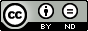### About Solution of the Nonlinear Generalized Abel Integral Equation

Burkhan Kalimbetov

#### Abstract

As is known, many problems of electronics, nuclear physics, optics and astrophysics, etc. are described by the Abel integral equation of the first kind. In this paper we consider the nonlinear generalized Abel equation and show that its solution can be represented as an integral of a power function. It is shown that the constructed analytical solution and the symbolic solution obtained by means of the computer mathematics system Maple coincides, and their planar and spatial graphs are presented.

#### Keywords

integral equation, Abel integral equation, tautochron problem, improper integral, Euler integral, computer mathematics system Maple.

PDF

#### References

Aladiev, V. (2006) Fundamentals of Programming in Maple, Tallinn.

Fikhtengolts, G. (1969) Course of Differential and Integral Calculus, Vol. II, Moscow, Nauka.

R. Gorenflo, R. and S. Vessella, S. (1991) Abel integral equations, Lecture Notes in Mathematics, 1461, Springer, Berlin.

Kosarev, E. (1980) Applications of integral equations of the first kind in experiment physics, Comput. Phys. Commun. 20, 69–75.

Muintz, G. (1934) Integral Equations. Part one. Linear Volterra equations, Moscow, GTTI.

]6] Plato, R. (2005) Fractional multistep methods for weakly singular Volterra integral equations of the first kind with perturbed data, Numer. Funct. Anal. Optim. 26, 249–269.

DOI: https://doi.org/10.24203/ajfam.v7i1.5620

### Refbacks

• There are currently no refbacks.

Copyright (c) 2019 Asian Journal of Fuzzy and Applied MathematicsThis work is licensed under a Creative Commons Attribution-NoDerivatives 4.0 International License.